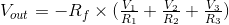## Working principle

A summing amplifier is an example of an inverting amplifier with multiple inputs, enabling to effectively add several individual input signals, which proves to be useful in audio mixing applications. Its output is the sum of each input scaled by the corresponding resistor ratio:### Experiments

• To create an averaging amplifier, all input resistors must be of equal value and the ratio of any of those resistors to Rf needs to be equal to the number of inputs. To achieve this, equate R1, R2 and R3 to 3 ohms (leave Rf at 1 ohm) and observe the output, which should now equal to the negative of the average of all input voltages.
• Equate all resistor values to create an adder configuration. What is the output voltage in terms of the input voltages now?
• Make V2 and V3 equal to zero and verify that the amplifier now behaves like an inverting amplifier with just one input (V1).